Previous: Mesh location Up: User Training Next: Physics

This is an automatically generated documentation by LaTeX2HTML utility. In case of any issue, please, contact us at info@cfdsupport.com.

## Test case: Cavity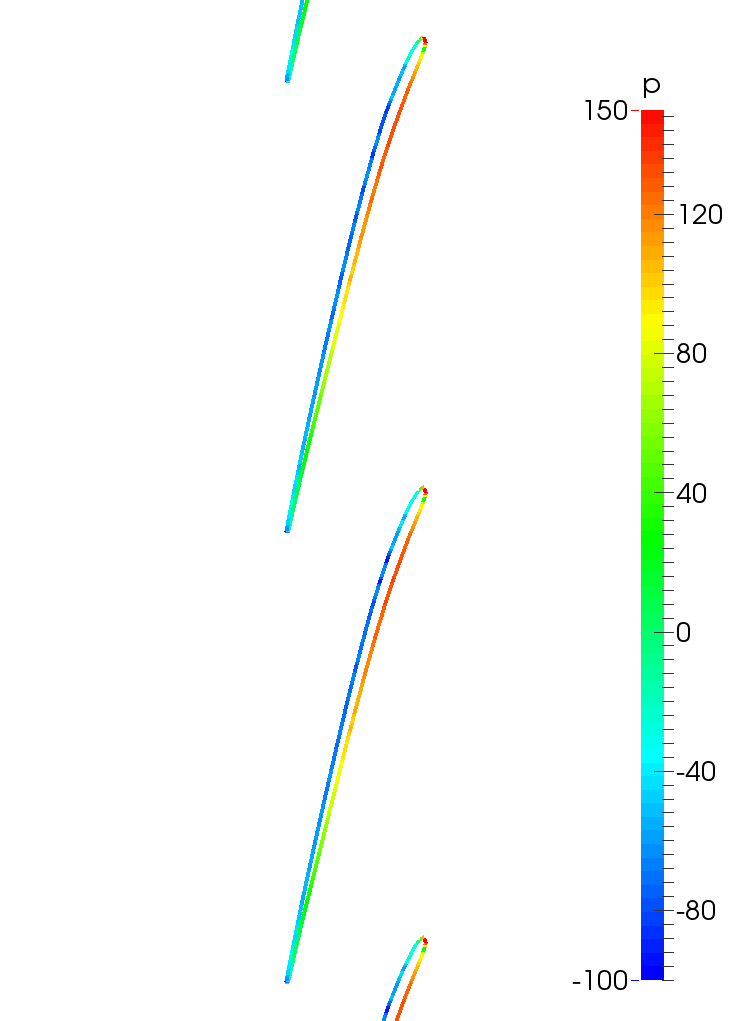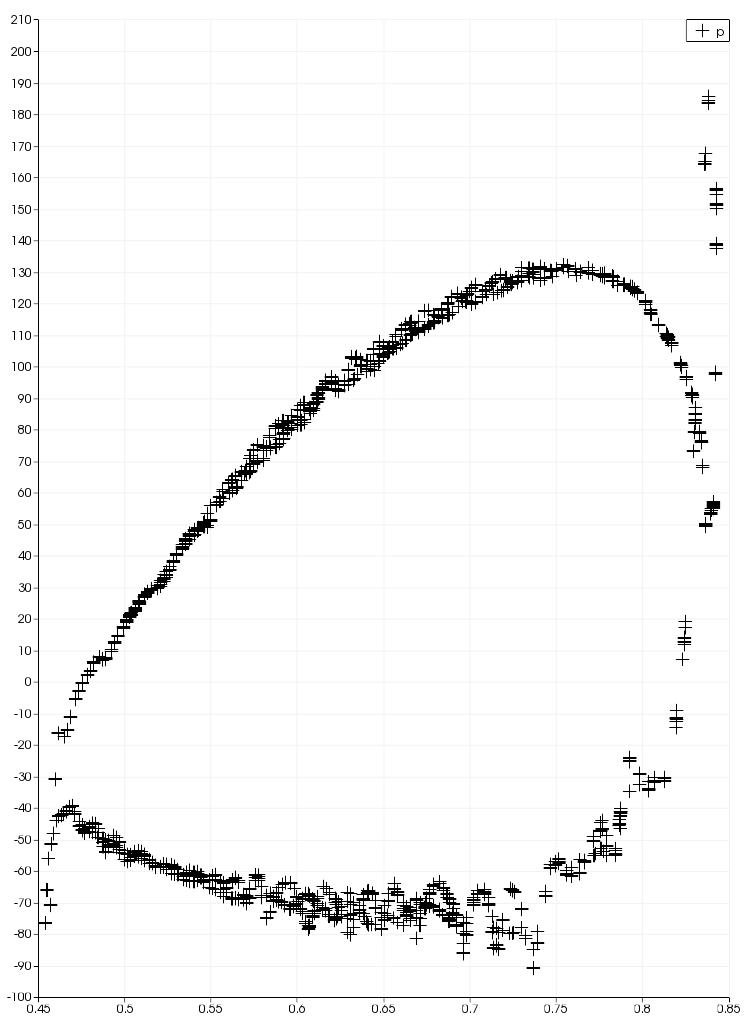Figure: Turbo Blade Post; Kaplan Turbine Tutorial; The unwrapped mesh – a wheel region. Colored by pressure; OpenFOAM Water Turbine CFD

## Fixed Static Pressure Family of Outlet Boundary Conditions

From mathematical point of view, fixed value boundary conditions are Dirichlet boundary conditions. NOTE: For incompressible fluid flow, all the pressure dimensions are always [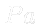/{rho}]=[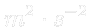], sometimes called kinematic pressure. So, all the pressures are divided by the density. Also all the mass flow rates, torques and powers are like divided by density. For incompressible fluid flow, the pressure value is alway relative, not absolute (this is why the zero value is often prescribed at the outlet).

Previous: Simulation Up: TCFD – GUI Setup Next: Reference frames
This is an automatically generated documentation by LaTeX2HTML utility. In case of any issue, please, contact us at info@cfdsupport.com.

## Physics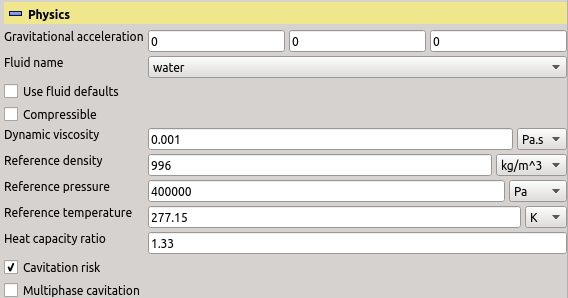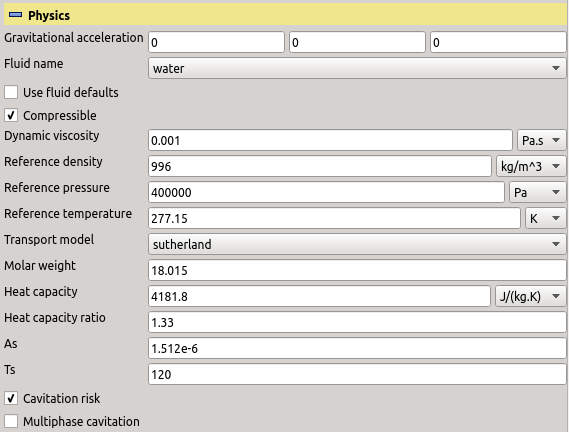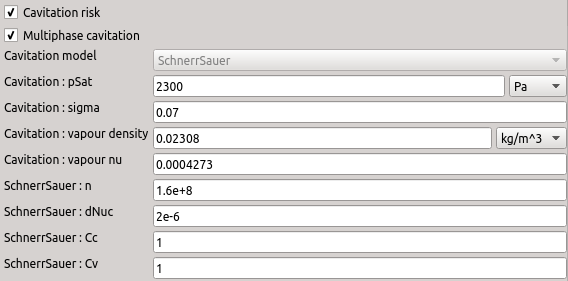Figure: Plugin TCFDSource – physical settings: Incompressible (top) and compressible (middle) variants and fully enabled multiphase cavitation section (bottom).

• The entry “Gravitational acceleration” sets the components of the gravitational acceleration vector. For most applications the gravitational force is negligible and these components can be zero. For large hydro (water) turbines it may be important. The typical setup with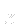axis pointing upwards would be the vector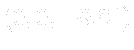.
• The drop-down list “Fluid name” offers selection of the fluid. This option has effect only with conjunction with “Use fluid defaults” or “Cavitation risk”.
• The switch “Use fluid defaults”, when checked, hides some further fields and assumes default values for them that are appropriate for the selected fluid at standard conditions. These are summarized in the table.
• The switch “Compressible” determines whether or not the fluid is considered compressible. When selected, then CFD Processor will also solve for the density and temperature fields, which are otherwise considered constant throughout the computational domain. The form automatically shows and hides entries that are relevant for the current choice.
• The value of “Dynamic viscosity” specifies the dynamic viscosity (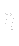) of the fluid. For incompressible cases it is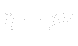, where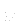is the kinematic viscosity.
• The value of “Reference density” is used for postprocessing of incompressible cases, where the density is not considered by the solver.
• The value of “Reference pressure” is used as a pressure value with respect to which all other pressures are measured. Typically, in incompressible simulation the user prescribes zero outlet pressure, while he or she actually means the ambient pressure. This entry then sets the ambient pressure.
• The value of “Reference temperature” is the value of the ambient temperature and is used for postprocessing of “Cavitation risk”.
• The list “Transport model” can be used to select the preferred transport (viscosity) model. There two models available: “constant” and “Sutherland”. When “Sutherland” transport model is selected, the entries “Ts” and “As” (parameters of the model) will show up. In contrast, when “constant” is selected, then one can set the Prandtl number “Pr”.
• The entry “Molar weight” is used only in compressible simulations to set up the thermophysical properties of the fluid. Molar weight is a standard chemical property of a substance.
• The entry “Heat capacity” is used only in compressible simulations to set up the thermophysical properties of the fluid. The constant-pressure heat capacity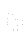is expected.
• The value of “Heat capacity ratio”,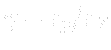, is used by the boundary condition “Total pressure” both in incompressible and compressible calculations. If that boundary condition is not used, this entry is ignored.
• The switch “Cavitation risk” can be used to request estimation of the cavitation. This switch is only available for “water” machines. The model was implemented according to following literature: , , . See the sectionfor details.
• The switch “Multiphase cavitation” will request use of a specialized cavitation solver for the transient phase of the calculation. When this switch is enabled, additional cavitation-related parameters can be set. Currently there is only one cavitation model available for this solver – the Schnerr-Sauer model . Multiphase cavitation is only available for water pumps and hydro turbines with transient calculation enabled. See the sectionfor details.
Table: Fluid defaults used when “Use fluid defaults” is checked.
 quantity symbol unit value (l)4-5 air water dynamic viscosity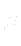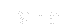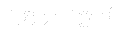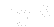reference density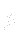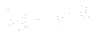1.2 998 molar weight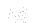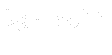28.9 18.015 heat capacity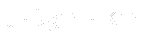1004 4181.8 Sutherland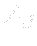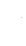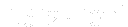Sutherland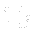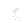120 120 Prandtl number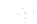0.7 7 heat capacity ratio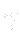1.4 1.33